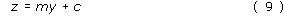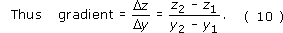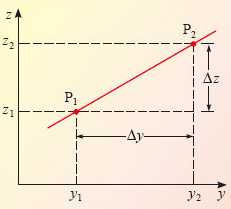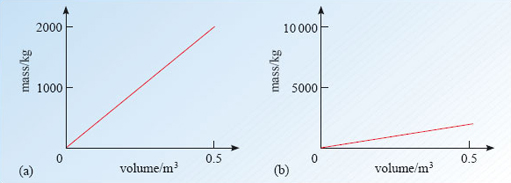Describing motion along a line

This free course is available to start right now. Review the full course description and key learning outcomes and create an account and enrol if you want a free statement of participation.

Free course

# 3.7 A note on straight-line graphs and their gradients

We end this section by reviewing some of the important features of straight-line graphs, though we do so in terms of two general variables z and y, rather than x and t, in order to emphasise their generality. If the graph of z against y is a straight line of the kind shown in Figure 22, then z and y are related by an equation of the formwhere m and c are constants. Here c represents the intercept of the graph and is equal to the value of z at which the plotted line crosses the z-axis (provided the z-axis passes through y = 0). The constant m represents the gradient of the graph and is obtained by dividing the change in z by the corresponding change in y, taking full account of the sign of each change.Figure 22: A straight-line graph of z against y

You may find it useful to remember that the gradient of a graph is given by its 'rise' over its 'run'.

There are several points to notice about this definition.

1. It applies only to straight-line graphs.

2. The units of the gradient are the units of z divided by the units of y.

3. The gradient of a straight-line graph is a constant (a number, multiplied by an appropriate unit). The same constant is obtained, no matter which two points (see P1 and P2 in Figure 22) are used to determine it.

4. The gradient of a straight-line graph can be positive, negative or zero. Equation 10 assigns a positive gradient to a graph sloping from bottom left to top right, as in Figure 22 for example, and a negative gradient to a graph sloping from top left to bottom right, as in Figure 15. A horizontal line has zero gradient.

Although the appearance of a graph can be changed by plotting the points on different scales (compare Figures 23a and b for example) the gradient, defined by Equation 10, is independent of the shape or size of the graph paper or display screen.Figure 23: Two graphs constructed from the same data but plotted with different scales. The gradients are the same, 4 ×103 kg m−3
S207_2

### Take your learning further

Making the decision to study can be a big step, which is why you'll want a trusted University. The Open University has 50 years’ experience delivering flexible learning and 170,000 students are studying with us right now. Take a look at all Open University courses.

If you are new to university level study, find out more about the types of qualifications we offer, including our entry level Access courses and Certificates.

Not ready for University study then browse over 900 free courses on OpenLearn and sign up to our newsletter to hear about new free courses as they are released.

Every year, thousands of students decide to study with The Open University. With over 120 qualifications, we’ve got the right course for you.

Request an Open University prospectus